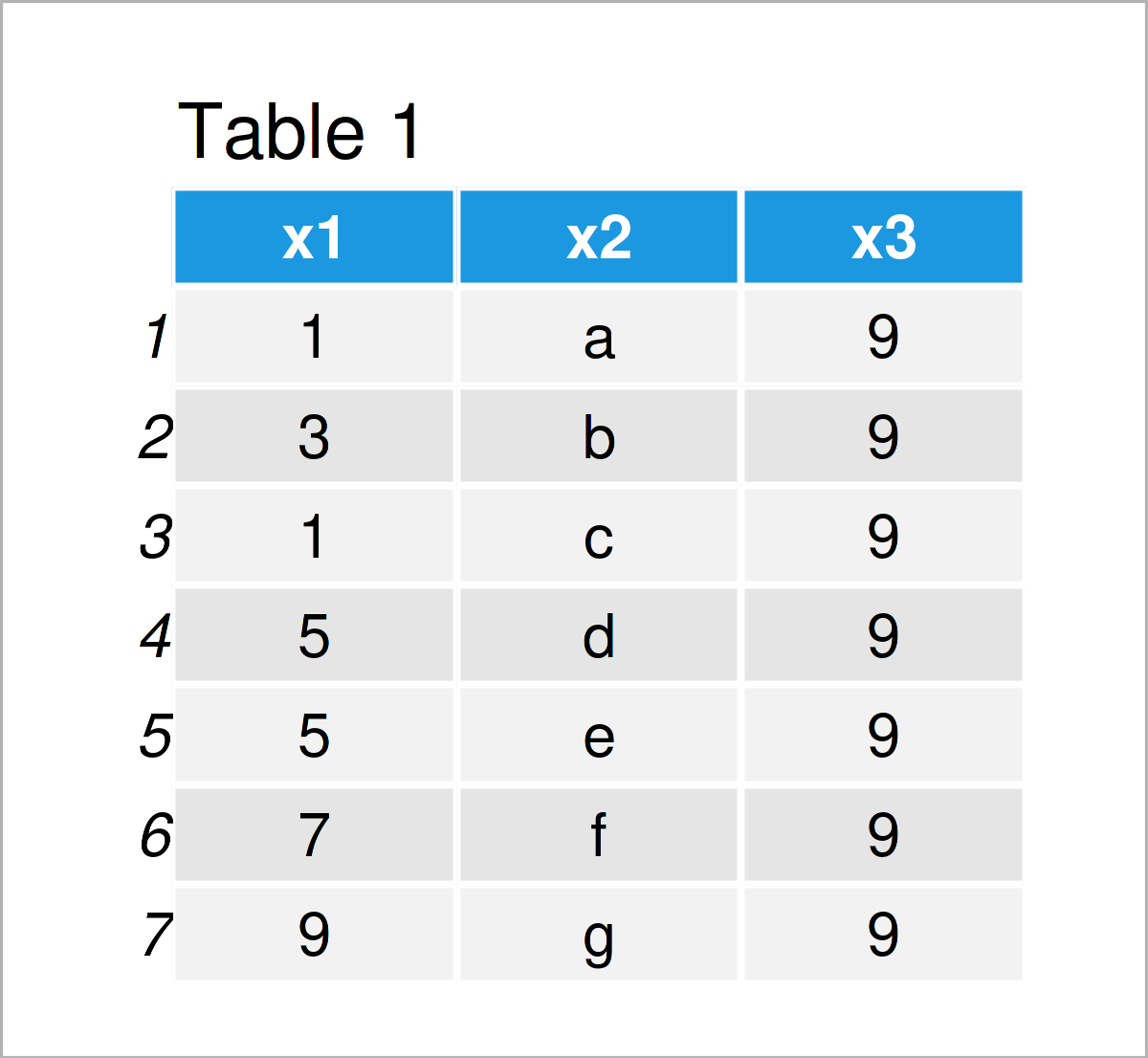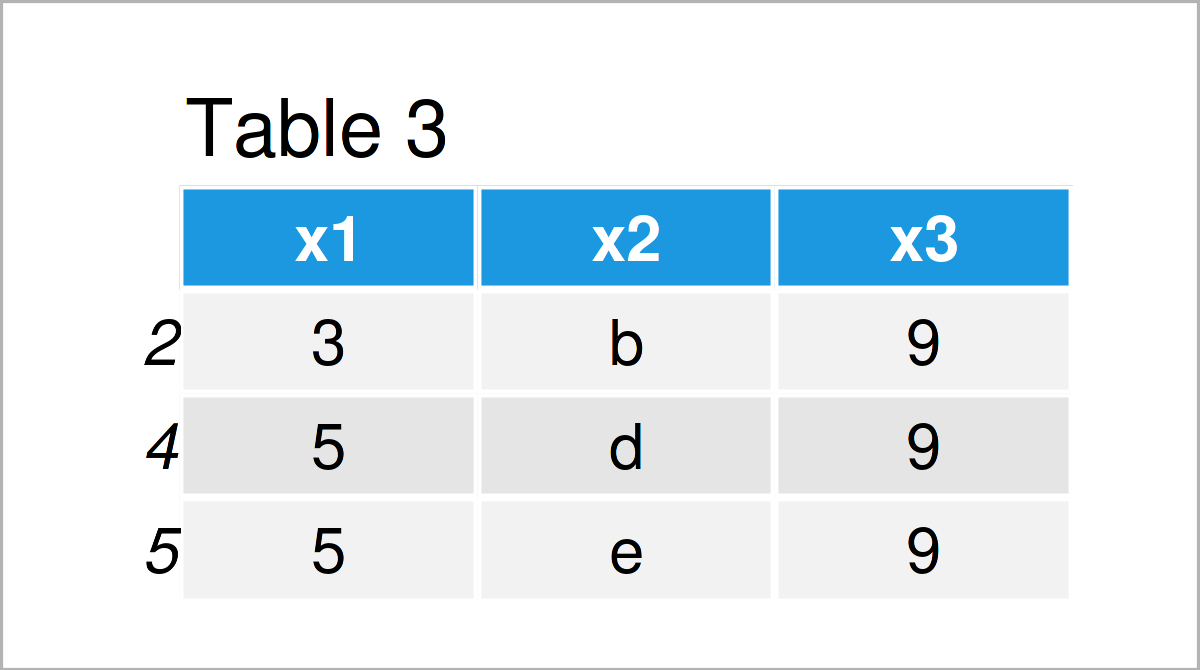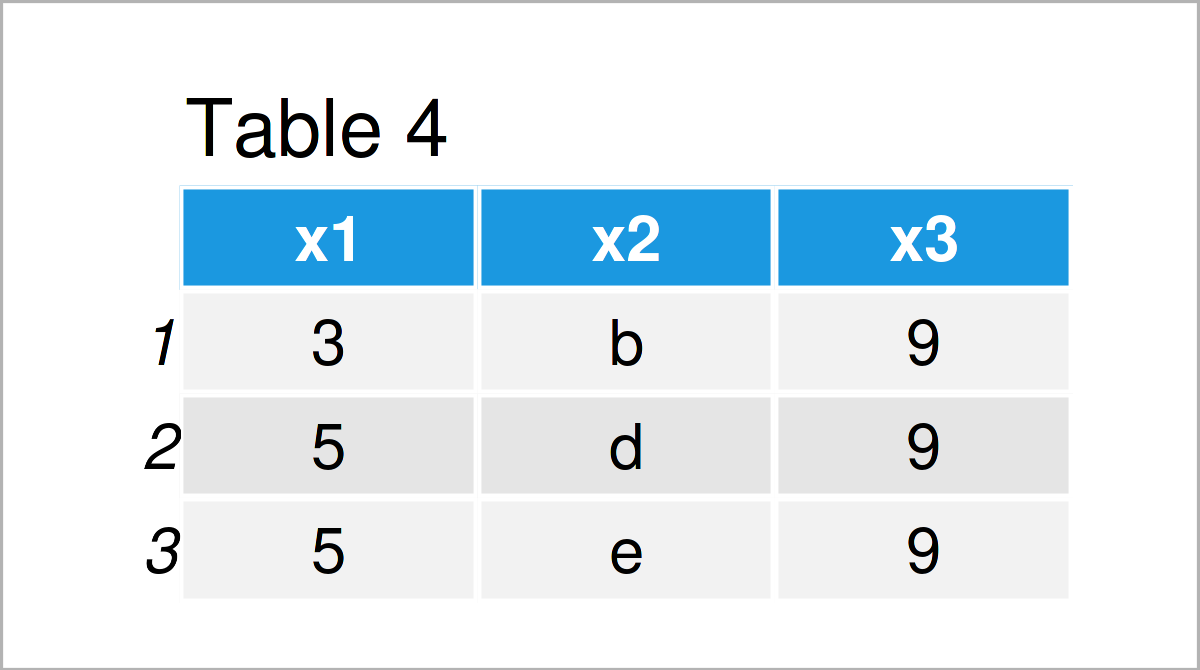# Filter Data Frame Rows Based On Range of Numbers in R (4 Examples)

This tutorial demonstrates how to subset rows of a data frame in a particular range of numbers in R.

The tutorial will consist of the following topics:

Let’s just jump right in:

## Creation of Example Data

I’ll use the following data as a basis for this R tutorial:

```data <- data.frame(x1 = c(1, 3, 1, 5, 5, 7, 9),    # Create example data frame
x2 = letters[1:7],
x3 = 9)
data                                               # Print example data frame```Table 1 shows the structure of our example data: It is constructed of seven rows and three columns.

## Example 1: Filter Data Frame Rows Based On Range of Numbers Using Square Brackets

Example 1 shows how to filter the rows of a data frame based on a range of numbers using square brackets and the %in% operator.

Consider the R code below:

```data_new1 <- data[data\$x1 %in% 3:5, ]              # Subset data frame
data_new1                                          # Print data frame subset```As shown in Table 2, the previous syntax has created a new data frame called data_new1 that contains only those rows where the column x1 contains integer values in the range from 3 to 5.

## Example 2: Filter Data Frame Rows Based On Range of Numbers Using subset() Function

This example shows how to use the subset function to select rows in a particular range of numbers.

Within the subset function, we are using the %in% operator once again:

```data_new2 <- subset(data, data\$x1 %in% 3:5)        # Subset data frame
data_new2                                          # Print data frame subset```In Table 3 it is shown that we have created the same data frame subset as in Example 1. However, this example has used the subset function instead of square brackets.

## Example 3: Filter Data Frame Rows Based On Range of Numbers Using filter() Function of dplyr Package

The following R code shows how to use the functions of the dplyr package to extract and drop rows inside and outside a range of numbers.

To be able to use the functions of the dplyr package, we first need to install and load dplyr:

```install.packages("dplyr")                          # Install & load dplyr package
library("dplyr")```

Next, we can apply the filter function in combination with the %in% operator to subset our data frame:

```data_new3 <- data %>%                              # Subset data frame
dplyr::filter(x1 %in% 3:5)
data_new3                                          # Print data frame subset```The output of the previous R syntax is shown in Table 4: Once again we have created the same data frame subset.

## Example 4: Filter Data Frame Rows Based On Range of Numbers Using filter() & between() Functions of dplyr Package

Alternatively to the %in% operator, we can also use the between function of the dplyr package.

The difference of the between function and the %in% operator is that between can easily be applied to non-integer values.

The following R code returns all rows of our data frame where the column x1 lies in the interval between 3 and 5 – no matter if the values are integers or numerical values with digits after the decimal point.

```data_new4 <- data %>%                              # Subset data frame
dplyr::filter(dplyr::between(x1, 3, 5))
data_new4                                          # Print data frame subset```As shown in Table 5, we have created another data frame subset. In this specific case, the output is the same as in the previous examples.

However, if our data frame would contain non-integer values in the range between 3 and 5 in the column x1, the resulting data frame subset would be different.

## Video, Further Resources & Summary

Do you need more info on the contents of this tutorial? Then you may watch the following video on my YouTube channel. In the video, I’m explaining the topics of the present article.

Furthermore, you might want to read the related articles on my website. I have published several other tutorials already.

In summary: At this point you should have learned how to select rows of a data frame in a particular range of numbers in a certain column in the R programming language. Don’t hesitate to kindly let me know in the comments below, in case you have any further questions.

Subscribe to the Statistics Globe Newsletter